• 如在PCA主成分分析中，需要计算样本的散度矩阵，有的论文是计算协方差矩阵。实质上二者意义差不多，散布矩阵（散度矩阵）前乘以系数1/(n-1)就可以得到协方差矩阵了。 在模式识别的教程中，散布矩阵也称为散度矩阵，...
协方差矩阵和散布矩阵的意义    【尊重原创，转载请注明出处】http://blog.csdn.net/guyuealian/article/details/68922981      在机器学习模式识别中，经常需要应用到协方差矩阵C和散布矩阵S。如在PCA主成分分析中，需要计算样本的散度矩阵，有的论文是计算协方差矩阵。实质上二者意义差不多，散布矩阵（散度矩阵）前乘以系数1/(n-1)就可以得到协方差矩阵了。   在模式识别的教程中，散布矩阵也称为散度矩阵，有的也称为类内离散度矩阵或者类内离差阵，用一个等式关系可表示为：  关系：散度矩阵=类内离散度矩阵=类内离差阵=协方差矩阵×（n-1）    样本的协方差矩阵乘以n-1倍即为散布矩阵，n表示样本的个数，散布矩阵的大小由特征维数d决定，是一个为d×d 的半正定矩阵。一、协方差矩阵的基础   对于二维随机变量（X,Y）之间的相互关系的数字特征，我们用协方差来描述，记为Cov(X,Y)：那么二维随机变量(X,Y)的协方差矩阵，为：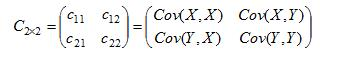对于三维随机变量X=（X1, X2, X3）的协方差矩阵可表示为：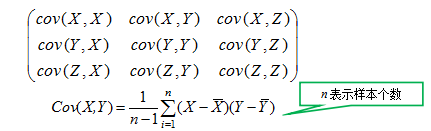对于n维X=（X1, X2....X n）协方差矩阵：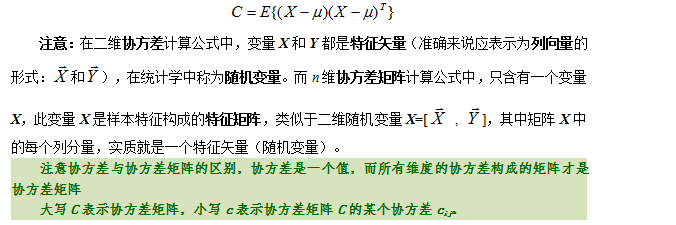说明：     （1）协方差矩阵是一个对称矩阵，且是半正定矩阵，主对角线是各个随机变量 的方差（各个维度上的方差）。   （2）标准差和方差一般是用来描述一维数据的；对于多维情况，而协方差是用于描述任意两维数据之间的关系，一般用协方差矩阵来表示。因此协方差矩阵计算的是不同维度之间的协方差，而不是不同样本之间的。   （3）协方差计算过程可简述为：先求各个分量的均值E(Xi)和E(Xj)，然后每个分量减去各自的均值得到两条向量，在进行内积运算，然后求内积后的总和，最后把总和除以n-1。例子：设有8个样本数据，每个样本有2个特征：(1,2);(3 3);(3 5);(5 4);(5 6);(6 5);(8 7);(9 8)，那么可以看作二维的随机变量（X,Y），即   X =[1 3 3 5 5 6 8 9]   Y =[2 3 5 4 6 5 7 8]   Matlab中可以使用cov(X, Y)函数计算样本的协方差矩阵，其中X,Y都是特征向量。当然若用X 表示样本的矩阵（X中每一行表示一个样本，每列是一个特征），那么可直接使用cov(X)计算了。clear all
clc
X=[1,2;3 3;3 5;5 4;5 6;6 5;8 7;9 8]%样本矩阵：8个样本，每个样本2个特征
covX= cov(X)%使用cov函数求协方差矩阵运行结果为：covX =

7.1429    4.8571
4.8571    4.0000当然，可以按定义计算，Matlab代码如下：clear all
clc
X=[1,2;3 3;3 5;5 4;5 6;6 5;8 7;9 8] %样本矩阵：8个样本，每个样本2个特征
covX= cov(X)                                %使用cov函数求协方差矩阵
%% 按定义求协方差矩阵：（1）使用分量的方法，先求协方差，再组合成协方差矩阵
meanX=mean(X)        %样本均值
varX=var(X)               %样本方差
[Row Col]=size(X);
dimNum=Row;          %s样本个数size(X,1)=8
dim1=X(:,1);              %特征分量1
dim2=X(:,2);              %而在分量2
c11=sum( (dim1-mean(dim1)) .* (dim1-mean(dim1)) ) / ( dimNum-1 );
c21=sum( (dim2-mean(dim2)) .* (dim1-mean(dim1)) ) / ( dimNum-1 );
c12=sum( (dim1-mean(dim1)) .* (dim2-mean(dim2)) ) / ( dimNum-1 );
c22=sum( (dim2-mean(dim2)) .* (dim2-mean(dim2)) ) / ( dimNum-1 );
C22=[c11,c12;c21,c22]%协方差矩阵

%% 或者（2）直接求协方差矩阵：
tempX= repmat(meanX,Row,1);
C22=(X-tempX)'*(X-tempX)/(dimNum-1)运行结果：covX =

7.1429    4.8571
4.8571    4.0000

meanX =

5     5

varX =

7.1429    4.0000

C22 =

7.1429    4.8571
4.8571    4.0000

C22 =

7.1429    4.8571
4.8571    4.0000说明：从中可以发现，样本的协方差矩阵的对角线即为样本的方差。二、协方差矩阵的意义   为了更好理解协方差矩阵的几何意义，下面以二维正态分布图为例（假设样本服从二维正态分布）：clear all;clc
mu=[0,0];         % 均值向量
C=[5 0;0 1]       %样本的协方差矩阵
[V,D] =eigs(C)    %求协方差矩阵的特征值D和特征向量V
%% 绘制二维正态分布图
[X,Y]=meshgrid(-10:0.3:10,-10:0.3:10);%在XOY面上，产生网格数据
p=mvnpdf([X(:) Y(:)],mu,C);%求取联合概率密度，相当于Z轴
p=reshape(p,size(X));%将Z值对应到相应的坐标上
figure
set(gcf,'Position',get(gcf,'Position').*[1 1 1.3 1])
subplot(2,3,[1 2 4 5])
surf(X,Y,p),axis tight,title('二维正态分布图')
subplot(2,3,3)
surf(X,Y,p),view(2),axis tight,title('在XOY面上的投影')
subplot(2,3,6)
surf(X,Y,p),view([0 0]),axis tight,title('在XOZ面上的投影');协方差矩阵C的特征值D和特征向量V分别为：V =
1     0
0     1

D =
5     0
0     1说明：   1)均值[0,0]代表正态分布的中心点，方差代表其分布的形状。   2)协方差矩阵C的最大特征值D对应的特征向量V指向样本分布的主轴方向。例如，最大特征值D1=5对应的特征向量V1=[1 0]T即为样本分布的主轴方向（一般认为是数据的传播方向）。次大特征值D2=1对应的特征向量V2=[0 1]T,即为样本分布的短轴方向。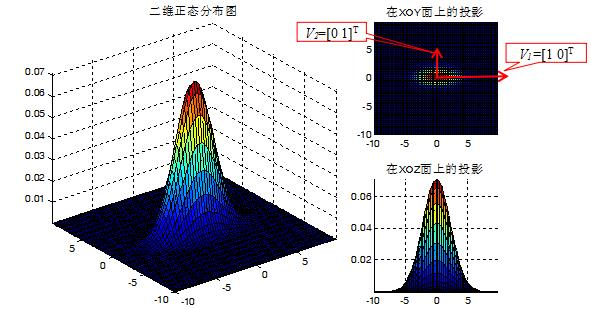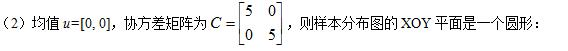协方差矩阵C的特征值D和特征向量V分别为：V =
0     1
1     0

D =

5     0
0     5 说明：   1)由于协方差矩阵C具有两个相同的特征值D1=D2=5,因此样本在V1和V2特征向量方向的分布是等程度的，故样本分布是一样圆形。   2)特征值D1和D2的比值越大，数据分布形状就越扁；当比值等于1时，此时样本数据分布为圆形。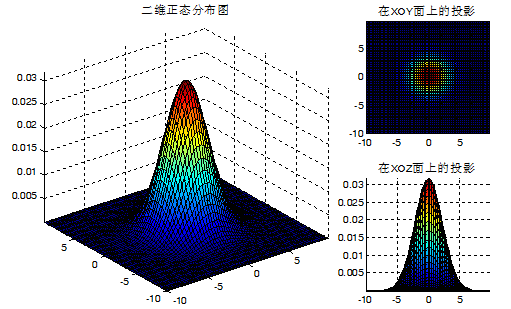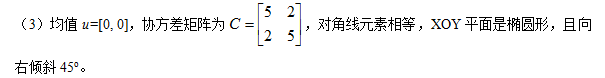协方差矩阵C的特征值D和特征向量V分别为：V =
0.7071   -0.7071
0.7071    0.7071

D =
6     0
0     4 说明：   1)特征值的比值D1/D2=6/4=1.5>1，因此样本数据分布形状是扁形，数据传播方向（样本的主轴方向）为V1=[0.7071 0.7071]T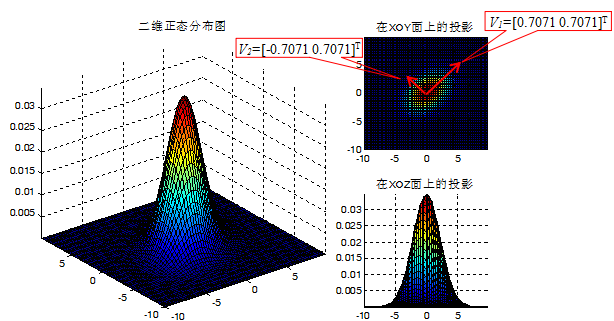综合上述，可知： (1)样本均值决定样本分布中心点的位置。(2)协方差矩阵决定样本分布的扁圆程度。   是扁还是圆，由协方差矩阵的特征值决定：当特征值D1和D2的比值为1时（D1/D2=1），则样本分布形状为圆形。当特征值的比值不为1时，样本分布为扁形；   偏向方向（数据传播方向）由特征向量决定。最大特征值对应的特征向量，总是指向数据最大方差的方向（椭圆形的主轴方向）。次大特征向量总是正交于最大特征向量（椭圆形的短轴方向）。三、协方差矩阵的应用    协方差矩阵（散布矩阵）在模式识别中应用广泛，最典型的应用是PCA主成分分析了，PCA主要用于降维，其意义就是将样本数据从高维空间投影到低维空间中，并尽可能的在低维空间中表示原始数据。这就需要找到一组最合适的投影方向，使得样本数据往低维投影后，能尽可能表征原始的数据。此时就需要样本的协方差矩阵。PCA算法就是求出这堆样本数据的协方差矩阵的特征值和特征向量，而协方差矩阵的特征向量的方向就是PCA需要投影的方向。    关于PCA的原理和分析，请见鄙人的博客：    《PCA主成分分析原理分析和Matlab实现方法》：http://blog.csdn.net/guyuealian/article/details/68487833如果你觉得该帖子帮到你，还望贵人多多支持，鄙人会再接再厉，继续努力的~展开全文协方差矩阵 散布矩阵 协方差矩阵的意义
• 在机器学习模式识别中，经常需要应用到协方差矩阵C 和散度 矩阵S。如在PCA(Principal ...在模式识别中，散度矩阵也称为 散步矩阵，有的也称为类内离 散度矩阵或者类内离差阵，用一个等式关系可表示为： 散度...
在机器学习模式识别中，经常需要应用到协方差矩阵C和散度矩阵S。如在PCA(Principal Component Analysis)主成分分析中，需要计算样本的散度矩阵，有的论文是计算协方差矩阵。实质上两者意义差不多，散度矩阵乘以1/(n-1)就可以得到协方差矩阵了。
在模式识别中，散度矩阵也称为散步矩阵，有的也称为类内离散度矩阵或者类内离差阵，用一个等式关系可表示为：

散度矩阵=类内离散度矩阵=类内离差阵=协方差矩阵 × (n-1)

样本的协方差矩阵乘以n-1倍等于散度矩阵，其中n表示样本个数，散度矩阵的大小由特征维数d决定，是一个d×d的半正定矩阵。
一、协方差矩阵基础
对于二维随机变量（X，Y）之间的相互关系的数字特征，我们用协方差来描述，记为Cov（X，Y）：
$Cov(X,Y)=E\left \{ [X-E(X)][Y-E(Y)]\right \}=E(XY)-E(X)E(Y)$
$Cov(X,Y)=\frac{1}{n-1}\sum _{i=1}^{n}(x-\bar{X})(y-\bar{Y})$
那么二维随机变量（X，Y）的协方差矩阵为：
$C_{2\times 2}=\begin{pmatrix} C_{1\times 1} &C_{1\times 2} \\ C_{2\times 1} & C_{2\times 2} \end{pmatrix}=\begin{pmatrix} Cov(X,X) &Cov(X,Y) \\ Cov(Y,X)& Cov(Y,Y) \end{pmatrix}$
同理，对于三维随机变量（X，Y，Z）的协方差矩阵可表示为：对于n维$X=(X_{1},X_{2},...,X_{n})$协方差矩阵：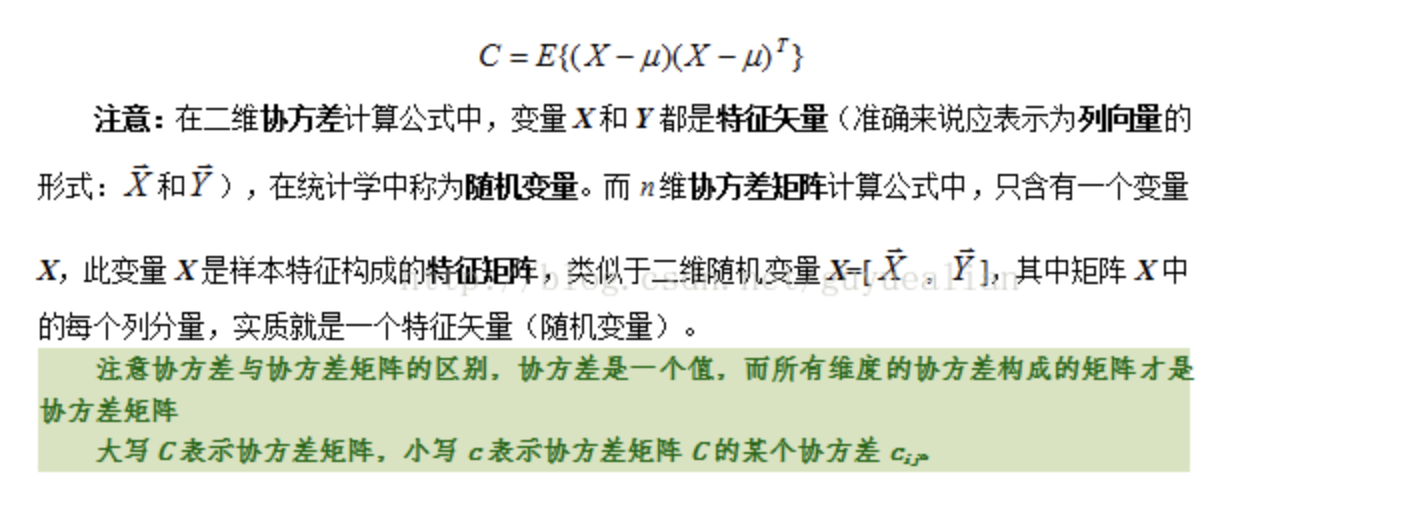说明：

协方差矩阵是一个对称矩阵，且是半正定矩阵，主对角线是各个随机变量的方差（各个特征维度上的方差）
标准差和方差一般是用来描述一维数据的；对于多维情况，协方差是用于描述任意二维数据之间的关系（即两个特征之间的关系），一般用协方差矩阵来表示。因此协方差矩阵计算的是不同维度（不同特征）之间的协方差，而不是不同样本之间的。
协方差计算过程可简述为：先求各个分量的均值$E(X_{i}),E(X_{j})$,然后每个分量减去各自的均值得到两条向量，再进行内积运算，然后求内积后的总和，最后除以n-1.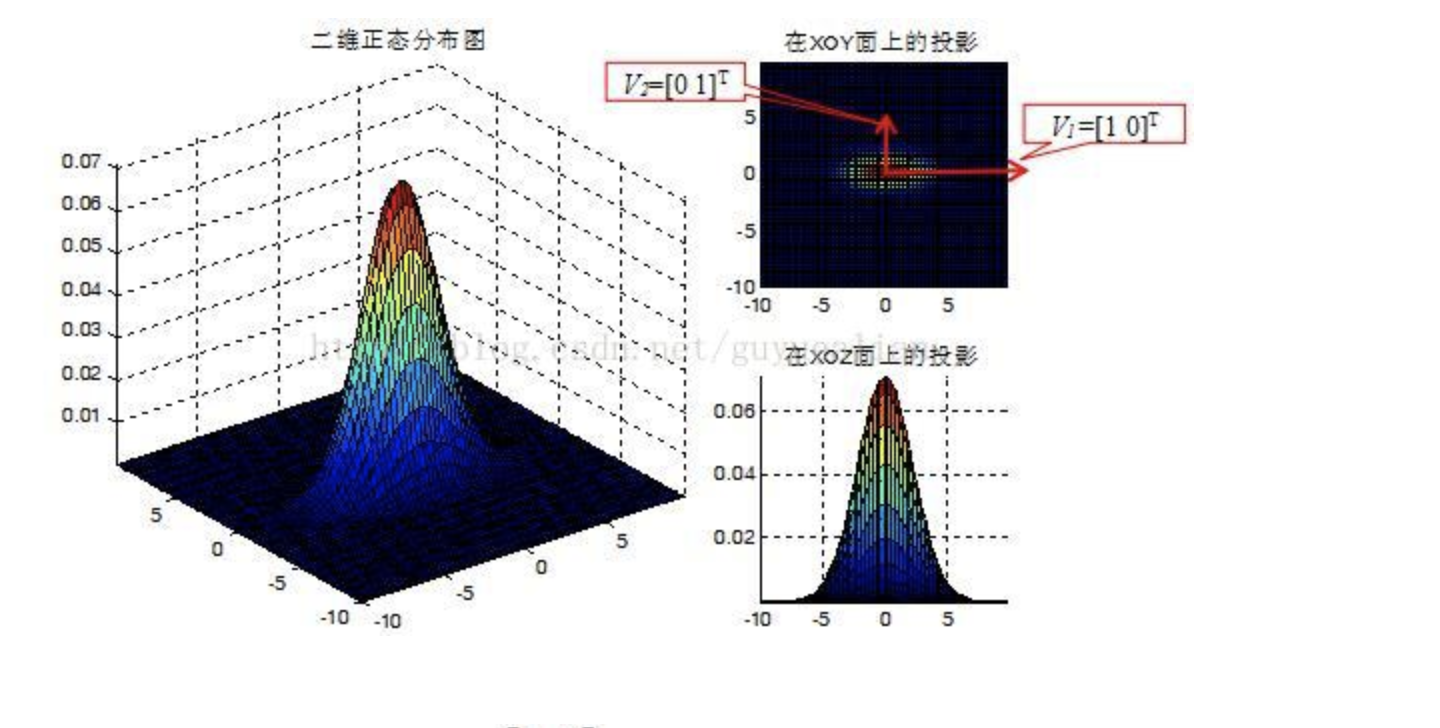二、协方差矩阵的意义
为了更好的理解协方差矩阵的几何意义，下面以二维正态分布图为例（假设样本服从二维正态分布）：协方差矩阵C的特征值D与特征向量V分别为：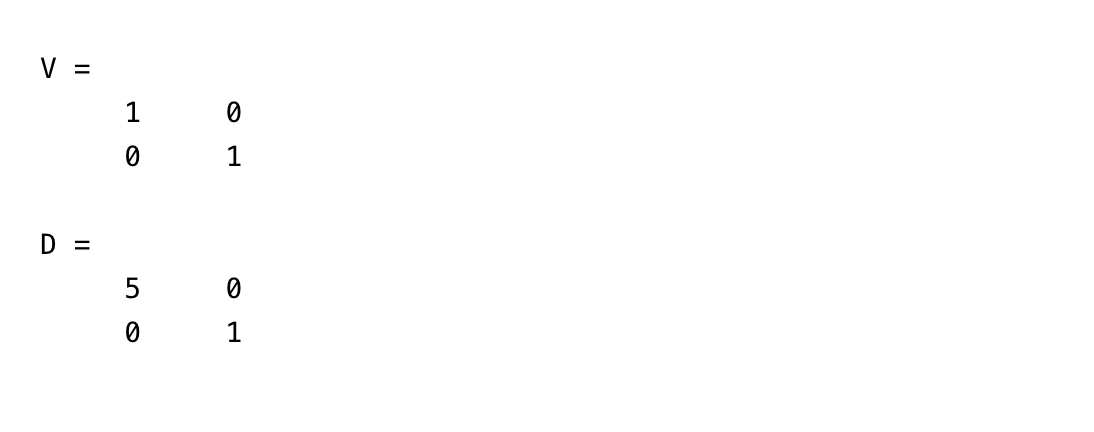说明：

均值[0,0]代表正态分布的中心点，方差代表其分布的形状。
协方差矩阵C的最大特征值D对应的特征向量V指向样本分布的主轴方向。例如，最大特征值$D_{1}=5$，其对应的特征向量$V_{1}=[1,0]^{T}$即为样本分布的主轴方向（一般认为是数据的传播方向）。次大特征值$D_{2}=1$所对应的特征向量$V_{2}=[0,1]^{T}$即为样本分布的短轴方向。

总之：

样本均值决定样本分布中心点的位置
协方差矩阵决定样本分布的扁圆程度

是扁还是圆，由协方差矩阵的特征值决定：当特征值D1和D2的比值为1（D1/D2=1）,则样本分布形状为圆形；当特征值的比值不为1时，样本分布为扁形。
偏向方向（数据传播方向）由特征向量决定。最大特征值对应的特征向量，总是指向数据最大方差的方向（椭圆形的主轴方向）。次大特征向量总是正交于最大特征向量（椭圆形的短轴方向）。

三、协方差矩阵的应用
协方差矩阵（散布矩阵）在模式识别中应用广泛，最典型的应用是PCA主成分分析了，PCA主要用于降维，其意义就是将样本数据从高维空间投影到低维空间中，并尽可能的在低维空间中表示原始数据。这就需要找到一组最合适的投影方向，使得样本数据往低维投影后，能尽可能表征原始的数据。此时就需要样本的协方差矩阵。PCA算法就是求出这堆样本数据的协方差矩阵的特征值和特征向量，而协方差矩阵的特征向量的方向就是PCA需要投影的方向!


展开全文• 类间散度矩阵: SB=(μ1−μ2)(μ1−μ2)T,(3)S_B=(μ_1-μ_2 ) (μ_1-μ_2 )^T,\tag3SB​=(μ1​−μ2​)(μ1​−μ2​)T,(3) 则欲最大化的目标可重写为 J=wTSBwwTSWw(4)J=\frac{w^T S_B w}{w^T S_W w}\tag4J=wTSW​...

LDA算法概述：
线性判别式分析(Linear Discriminant Analysis, LDA)，也叫做Fisher线性判别(Fisher Linear Discriminant ,FLD)，是模式识别的经典算法，它是在1996年由Belhumeur引入模式识别和人工智能领域的。线性鉴别分析的基本思想是将高维的模式样本投影到最佳鉴别矢量空间，以达到抽取分类信息和压缩特征空间维数的效果，投影后保证模式样本在新的子空间有最大的类间距离和最小的类内距离，即模式在该空间中有最佳的可分离性。因此，它是一种有效的特征抽取方法。使用这种方法能够使投影后模式样本的类间散布矩阵最大，并且同时类内散布矩阵最小。就是说，它能够保证投影后模式样本在新的空间中有最小的类内距离和最大的类间距离，即模式在该空间中有最佳的可分离性。

说白了就是想让数据降维之后, 同一类的数据靠紧一点, 不同类的离远一点.

为什么要用LDA
既然PCA已经是一种表现很好的数据降维的方法，LDA也是一种将数据降维的方法。那为什么还要有LDA呢？
PCA是一种无监督的数据降维方法，与之不同的是LDA是一种有监督的数据降维方法。我们知道即使在训练样本上，我们提供了类别标签，在使用PCA模型的时候，我们是不利用类别标签的，而LDA在进行数据降维的时候是利用数据的类别标签提供的信息的。
从几何的角度来看，PCA和LDA都是讲数据投影到新的相互正交的坐标轴上。只不过在投影的过程中他们使用的约束是不同的，也可以说目标是不同的。PCA是将数据投影到方差最大的几个相互正交的方向上，以期待保留最多的样本信息。样本的方差越大表示样本的多样性越好，在训练模型的时候，我们当然希望数据的差别越大越好。否则即使样本很多但是他们彼此相似或者相同，提供的样本信息将相同，相当于只有很少的样本提供信息是有用的。样本信息不足将导致模型性能不够理想。这就是PCA降维的目标：将数据投影到方差最大的几个相互正交的方向上。这种约束有时候很有用，比如在下面这个例子：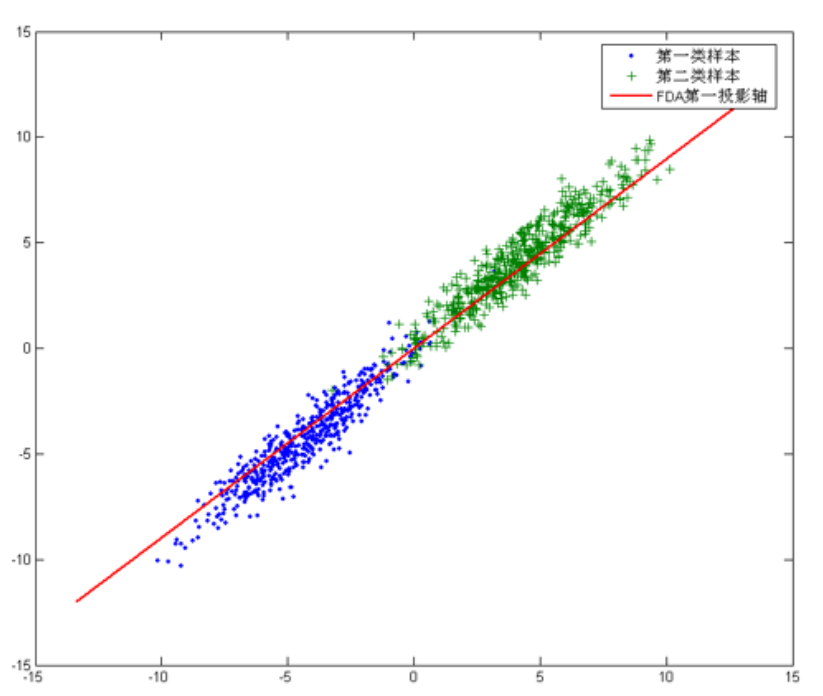对于这个样本集我们可以将数据投影到x轴或者y轴，但这都不是最佳的投影方向，因为这两个方向都不能最好地反映数据的分布。很明显还存在最佳的方向可以描述数据的分布趋势，那就是图中红色直线所在的方向。也是数据样本做投影，方差最大的方向。向这个方向做投影，投影后数据的方差最大，数据保留的信息最多。
但是，对于另外的一些不同分布的数据集，PCA的这个投影后方差最大的目标就不太合适了。比如对于下面图片中的数据集：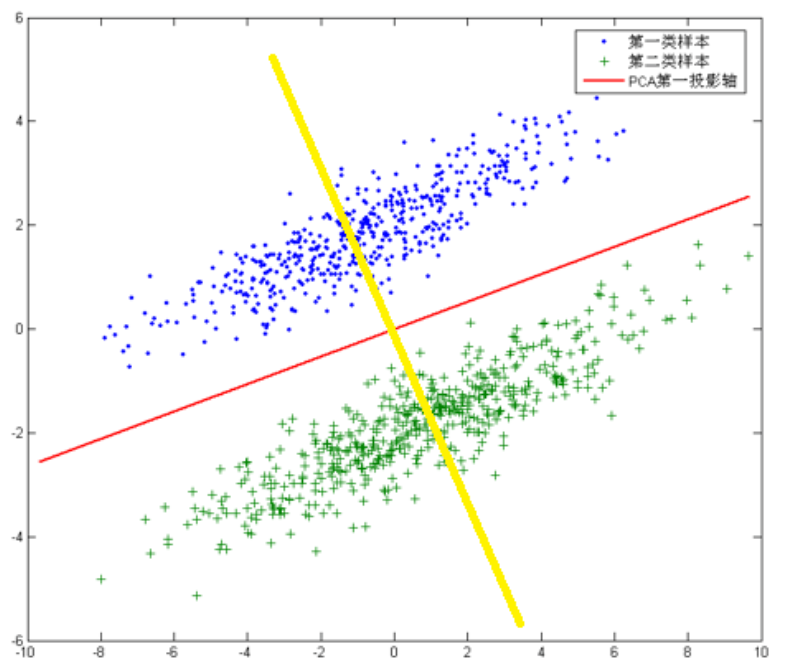针对这个数据集，如果同样选择使用PCA，选择方差最大的方向作为投影方向，来对数据进行降维。那么PCA选出的最佳投影方向，将是图中红色直线所示的方向。这样做投影确实方差最大，但是是不是有其他问题。聪明的你一定发现了，这样做投影之后两类数据样本将混合在一起，将不再线性可分，甚至是不可分的。这对我们来说简直就是地狱，本来线性可分的样本被我们亲手变得不再可分。
帅气英俊的你也一定发现了，图中还有一条耀眼的黄色直线，向这条直线做投影即能使数据降维，同时还能保证两类数据仍然是线性可分的。上面的这个数据集如果使用LDA降维，找出的投影方向就是黄色直线所在的方向。
这其实就是LDA的思想，或者说LDA降维的目标：将带有标签的数据降维，投影到低维空间同时满足三个条件：
尽可能多地保留数据样本的信息（即选择最大的特征是对应的特征向量所代表的的方向）。
寻找使样本尽可能好分的最佳投影方向。
投影后使得同类样本尽可能近，不同类样本尽可能远。
其实第二个和第三个条件是基本等价的，我们很容易想到，使样本尽可能好分的投影方向，就是要使投影后使得同类样本尽可能近，不同类样本尽可能远。
上面大致讲解的LDA的基本思想，以及与PCA的不同，下面就来介绍一下LDA模型。

符号说明：
$C:一个空间一共有C个类,$
$x∶表示训练样本，使用列向量表示$
$x_j^{(i)}:第i个类的第j个样本, 每个x_j^{(i)}是一个m行1列的矩阵，$
$M_i ∶表示第i类训练样本的数目（i=1,2,…,C）$
$M=\sum_{i=1}^CM_i ∶表示训练样本的总数目$
$\mu_i=\frac1{M_i}\sum_{j=1}^{M_i}X_j^{(i)}∶表示第i类训练样本的均值 （i=1,2,…,C）$
$\mu=\frac1{M}\sum_{i=1}^M x_i ∶表示全部训练样本的均值$
$w∶ 投影直线$
$\Sigma_i =\sum_{j=1}^{M_i}(x_j^{(i)}−\mu_i)(x_j^{(i)}−\mu_i)^T∶表示i类样本的协方差矩阵$
$p(i) ∶表示第i类的出现概率$
$p(i,j) ∶表示x_j^{(i)}的出现概率$
二类LDA原理:
由于是两类数据，因此我们只需要将数据投影到一条直线上即可。假设我们的投影直线是向量$w$,则对任意一个样本$x_i$,它在直线$w$的投影为$w^T x_i$,对于我们的两个类别的中心点$μ_1$,$μ_2$,在直线$w$的投影为$w^T μ_1$ 和$w^T μ_2$。由于LDA需要让不同类别的数据的类别中心之间的距离尽可能的大，也就是我们要最大化$||w^T μ_1-w^T μ_2 ||_2^2$,同时我们希望同一种类别数据的投影点尽可能的接近，也就是要同类样本投影点的协方差$w^T Σ_1 w$和$w^T Σ_2 w$尽可能的小，即最小化$w^T Σ_1 w+w^T Σ_2 w$。同时考虑二者，则可得到欲最大化的目标
$J=\frac{||w^T μ_1-w^T μ_2 ||_2^2}{w^T Σ_1 w+w^T Σ_2 w}=\frac{w^T (μ_1-μ_2 ) (μ_1-μ_2 )^T w}{w^T (Σ_1+Σ_2 )w} \tag1$
类内散度矩阵:
$S_W=Σ_1+Σ_2= ∑_{i=1}^{M_1}(x_1^{(i)}-μ_1 ) (x_1^{(i)}-μ_1)^T+ ∑_{i=1}^{M_2}(x_2^{(i)}-μ_2 ) (x_2^{(i)}-μ_2 )^T \tag2$
类间散度矩阵:
$S_B=(μ_1-μ_2 ) (μ_1-μ_2 )^T,\tag3$
则欲最大化的目标可重写为
$J=\frac{w^T S_B w}{w^T S_W w}\tag4$
不失一般性，令$w^T S_W w=1$，则优化目标等价于
$\max_w⁡ \quad w^T S_B w \qquad s.t. \quad w^T S_W w=1 \tag5$
由拉格朗日乘子法，上式等价于
$S_B w=λS_W w,\tag6$
其中λ是拉格朗日乘子. 注意到$S_B w$的方向恒为$μ_1-μ_2$, 不妨令
$S_B w=λ(μ_1-μ_2 ),\tag7$
代入式(6)中即得
$w=S_W^{-1} (μ_1-μ_2).\tag8$
考虑到数值解的稳定性，在实践中通常是对$S_W$进行奇异值分解，即$S_W=UΣV^T$，这里$Σ$是一个实对称矩阵，其对角线上的元素是$S_W$的奇异值，然后再由$S_W^{-1}=VΣ^{-1}U^T$得到$S_W^{-1}$，或者是令$S_W=S_W+γI$，$γ$是一个特别小的数，使得$S_W$可逆也是一种方法.
多类LDA原理:
在多类问题时，将$D$维的向量$x$投影到$d维的$y$.投影方程为:
$y=W^T x\tag9$
这里$W$是一个投影矩阵，每一个列向量$w_i$表示一个投影方向.
设数据集合为$X=\lbrace x_1^{(1)}，x_2^{(1)}，…，x_{M_1}^{(1)}，x_1^{(2)}，…x_{M_C}^{(C)}\rbrace$. 在多类的时候，过程与上面一样，不过优化的目标函数需要更改一下.
类内散度矩阵与二类时的定义一样，如下表示:
$S_W=∑_{i=1}^C∑_{j=1}^{M_i}p(i,j)(x_j^{(i)}-μ_i ) (x_j^{(i)}-μ_i )^T \tag{10}$
类间散度矩阵根据定义以及推导，得
\begin{aligned} S_B&=\frac12 ∑_{i=1}^C∑_{j=1}^Cp(i)p(j)(μ_i-μ_j ) (μ_i-μ_j )^T \\ &=\frac12 ∑_{i=1}^C∑_{j=1}^Cp(i)p(j)(μ_i μ_i^T-μ_i μ_j^T-μ_j μ_i^T+μ_j μ_j^T ) \\ &=\frac12 ∑_{i=1}^C{p(i) μ_i μ_i^T ∑_{j=1}^Cp(j)-p(i) μ_i ∑_{j=1}^Cp(j)μ_j^T- p(i)(∑_{j=1}^Cp(j)μ_j)μ_i^T- p(i) ∑_{j=1}^Cp(j)μ_j μ_j^T }\\ &=\frac12 ∑_{i=1}^C{p(i) μ_i μ_i^T-p(i) μ_i μ^T-p(i)μμ_i^T-p(i) ∑_{j=1}^Cp(j)μ_j μ_j^T }\\ &=\frac12 {∑_{i=1}^Cp(i) μ_i μ_i^T-μμ^T-μμ^T -∑_{j=1}^Cp(j)μ_j μ_j^T}\\ &=∑_{i=1}^Cp(i) (μ_i μ_i^T-μμ^T) \\ &=∑_{i=1}^Cp(i) (μ_i μ_i^T-μμ^T)+2∑_{i=1}^Cp(i)μμ^T-∑_{i=1}^Cp(i) μ_i μ^T- ∑_{i=1}^Cp(i)μμ_i^T \\ &=∑_{i=1}^Cp(i) (μ_i μ_i^T-μ_i μ^T-μμ_i^T+μμ^T)\\ &=∑_{i=1}^Cp(i)(μ_i-μ) (μ_i-μ)^T \end{aligned}
即“类间散度矩阵”可表示为:
$S_B=∑_{i=1}^Cp(i)(μ_i-μ) (μ_i-μ)^T \tag{11}$
同时也可以定义“总体散度矩阵”$S_T$，表示为:
$S_T=∑_{i=1}^C∑_{j=1}^{M_i}p(i,j)(x_i^{(j)}-μ) (x_i^{(j)}-μ)^T \tag{12}$
“类间散度矩阵”也可通过“总体散度矩阵”与“类内散度矩阵”的差值求出，即:
\begin{aligned} S_B&=S_T-S_W\\ &=∑_{i=1}^C∑_{j=1}^{M_i}p(i,j)(x_i^{(j)}-μ) (x_i^{(j)}-μ)^T -∑_{i=1}^C∑_{j=1}^{M_i}p(i,j)(x_i^{(j)}-μ_i ) (x_i^{(j)}-μ_i )^T \\ &=∑_{i=1}^C∑_{j=1}^{M_i}p(i,j)\lbrace(x_i^{(j)}-μ) (x_i^{(j)}-μ)^T-(x_i^{(j)}-μ_i ) (x_i^{(j)}-μ_i )^T \rbrace \\ &=∑_{i=1}^C\lbrace -p(i)μ_i μ^T- p(i)μμ_i^T+p(i)μμ^T+p(i)μ_i μ_i^T\rbrace \\ &=∑_{i=1}^Cp(i)(μ_i-μ) (μ_i-μ)^T \end{aligned}
得到$S_B$，进一步可以得到目标函数:
$J=\frac{|W^T S_B W|}{|W^T S_W W|} \tag{13}$
由于$S_B，S_T$不是标量，因此在目标函数中使用它们的行列式. 类似于二类推导的式子，可以得出
$S_W^{-1} S_B W=λW\tag{14}$
计算$S_W^{-1} S_B$的最大的$d$个特征值和对应的$d$个特征向量$(w_1,w_2,…,w_d)$,即可得到投影矩阵W.
总结一下LDA的实现过程:
1)计算每个类样本的均值向量$μ_i$和所有样本的均值向量$μ$。
2)计算类内散度矩阵$S_W$和类间散度矩阵$S_B$。
3)计算$S_W^{-1} S_B$的特征向量$W(w_1,w_2,…,w_D)$和对应的特征值$λ(λ_1,λ_2,…,λ_D)$。
4)选择$d$个最大特征值对应的矩阵$W_{m*d}$。
5)对数据集$X$进行降维，得到对应的降维数据集$Y=W^T X$。
等价模型
根据LDA的基本思想，即让不同类别的数据的类别中心之间的距离尽可能的大，同时希望同一种类别数据的投影点尽可能的接近，可以得到不同的优化函数，如:
$J(W)=\frac{|W^T S_B W|}{|W^T S_W W|}$
$J(W)=\frac{|W^T S_B W|}{|W^T S_T W|}$
$J(W)=\frac{|W^T (S_B-S_W )W|}{|W^T W|}$
以上优化目标的目的都是一样的，为了最大化类间距离同时最小化类内距离，根据以上不同优化目标函数能够得到不同的LDA模型。
正则化
让模型泛化性能更高，防止模型过拟合。也可为了让$S_W$一定可逆而加。
$J(W)=\frac{|W^T S_B W|}{|W^T (S_W+λI)W|}$
比较fisherface和eigenface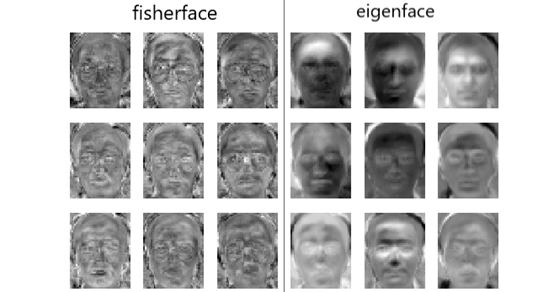LDA用于降维，和PCA有很多相同，也有很多不同的地方。
相同点：

两者均可以对数据进行降维。
两者在降维时均使用了矩阵特征分解的思想。

不同点：

LDA是有监督的降维方法，而PCA是无监督的降维方法
LDA降维最多降到类别数C-1的维数，而PCA没有这个限制。
LDA除了可以用于降维，还可以用于分类。
LDA选择分类性能最好的投影方向，而PCA选择样本点投影具有最大方差的方向。

参考
1.机器学习-线性判别分析.周志华
2.线性判别分析(LDA)


展开全文机器学习 人工智能
• 散度矩阵： 散布矩阵又称散度矩阵，将协方差矩阵乘以系数（n-1）就得到了散布矩阵，所有散布矩阵与协方差矩阵矩阵的作用是一样的，理解了协方差矩阵也就理解了散布矩阵，它们只有一个系数只差而已。 ...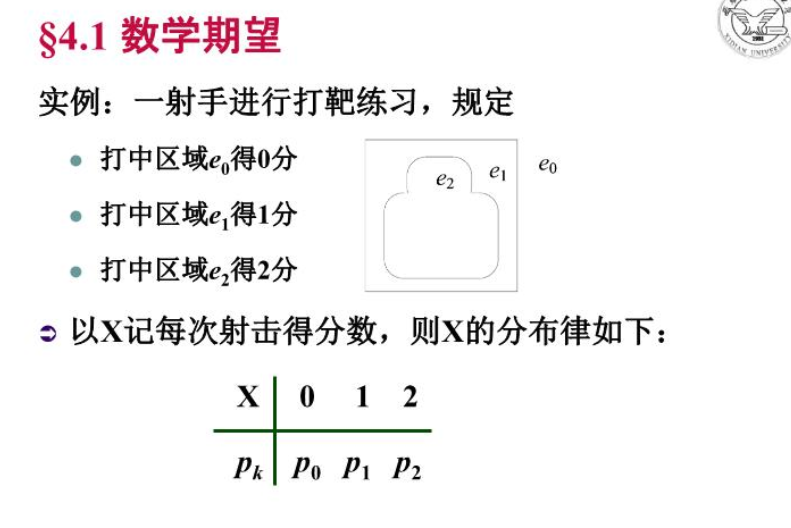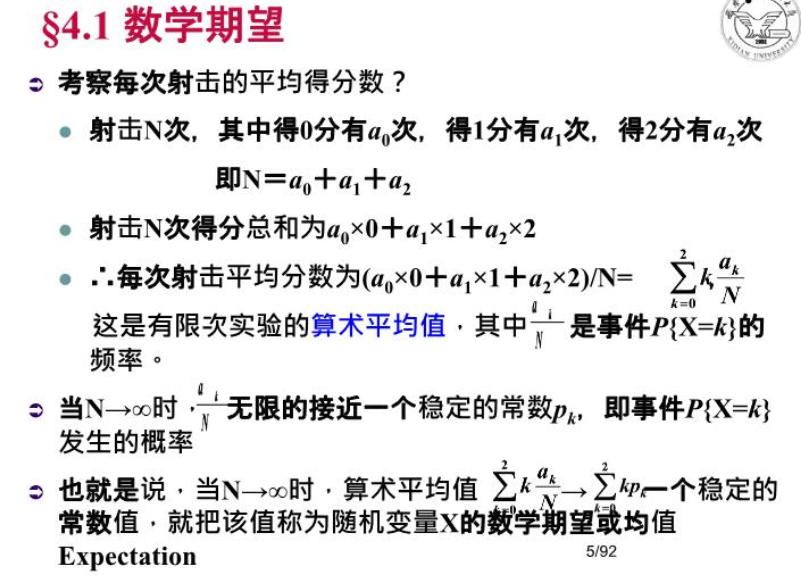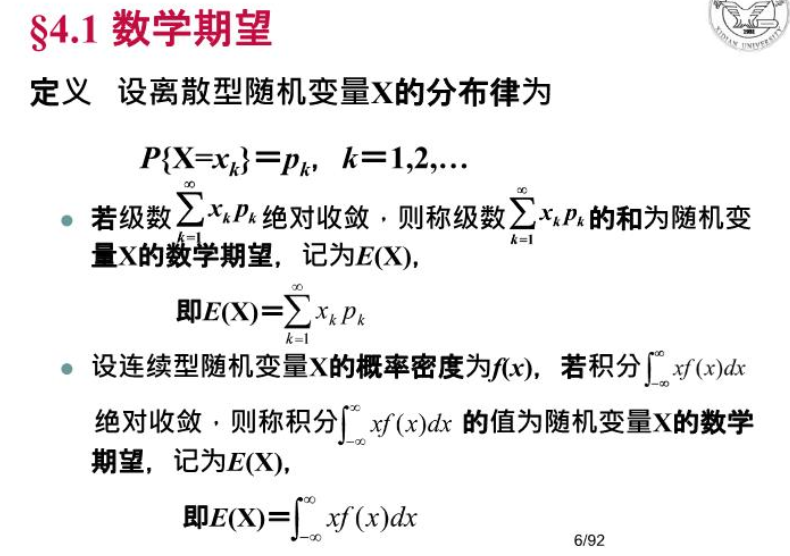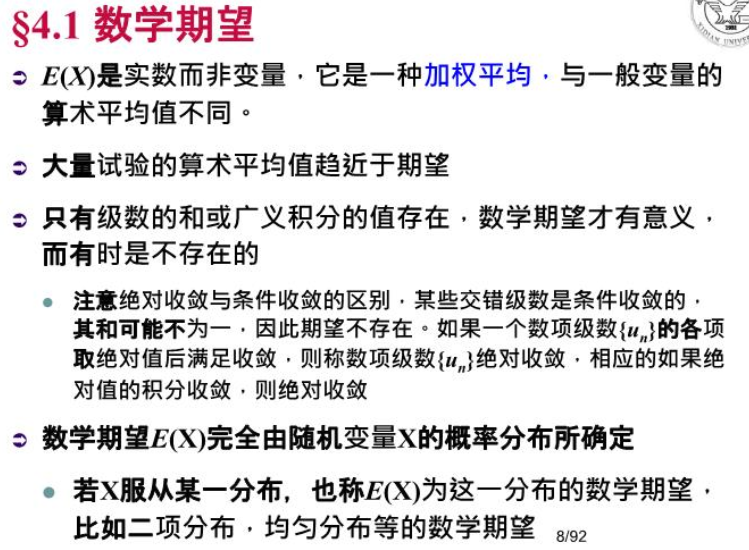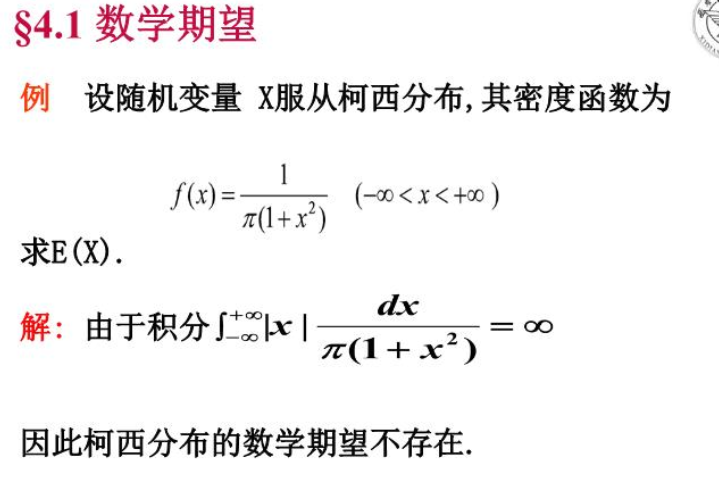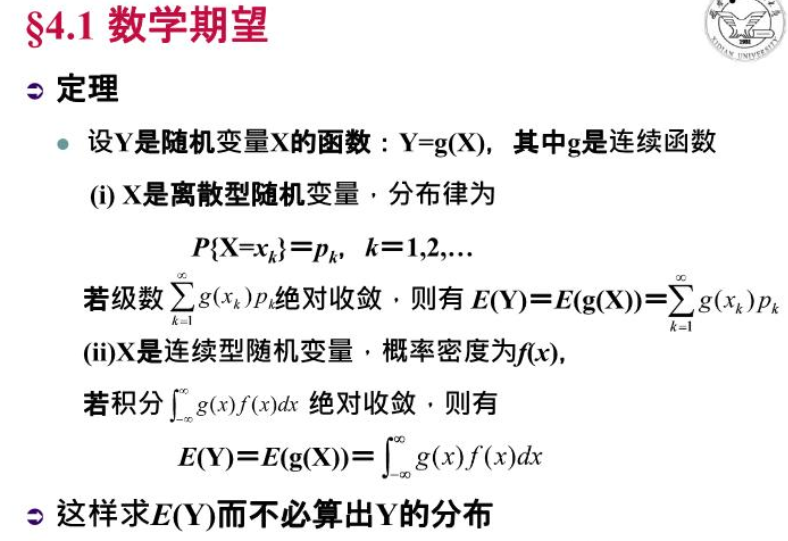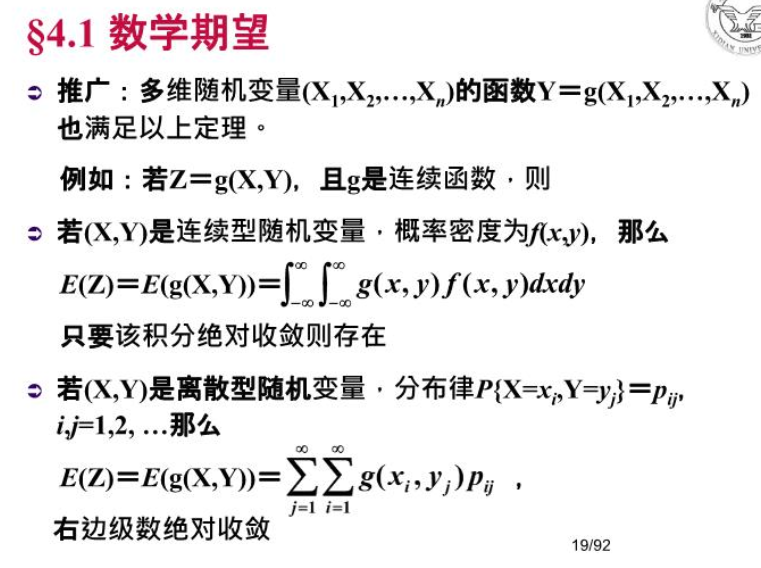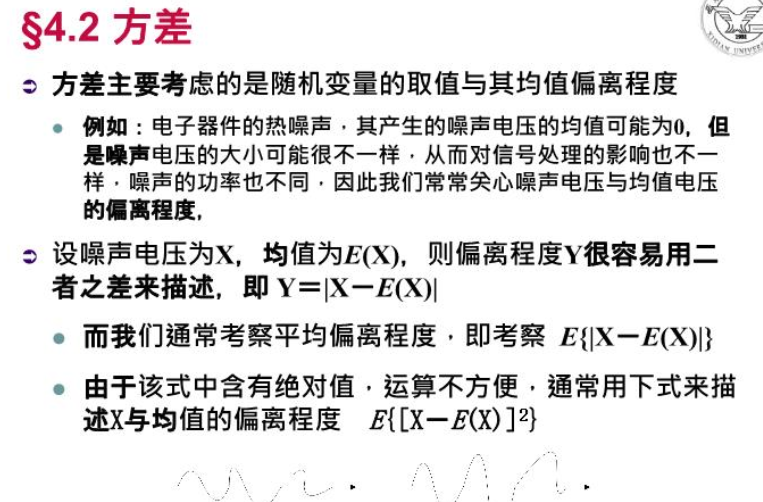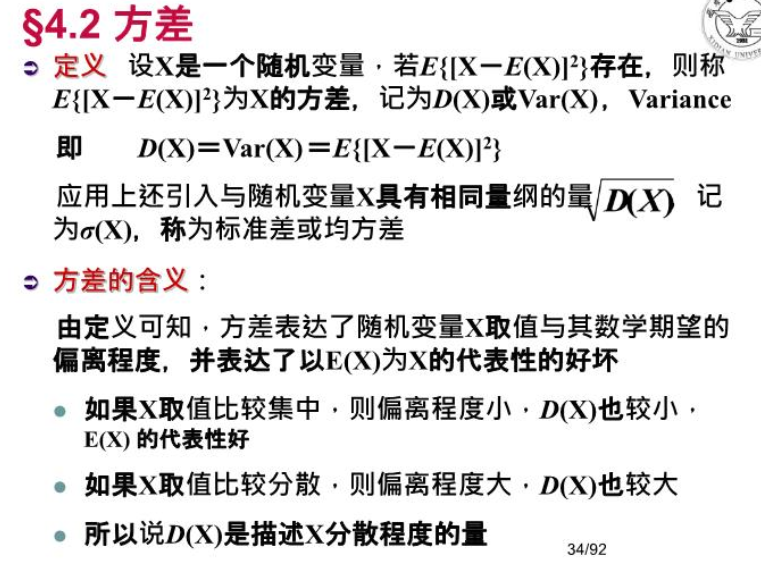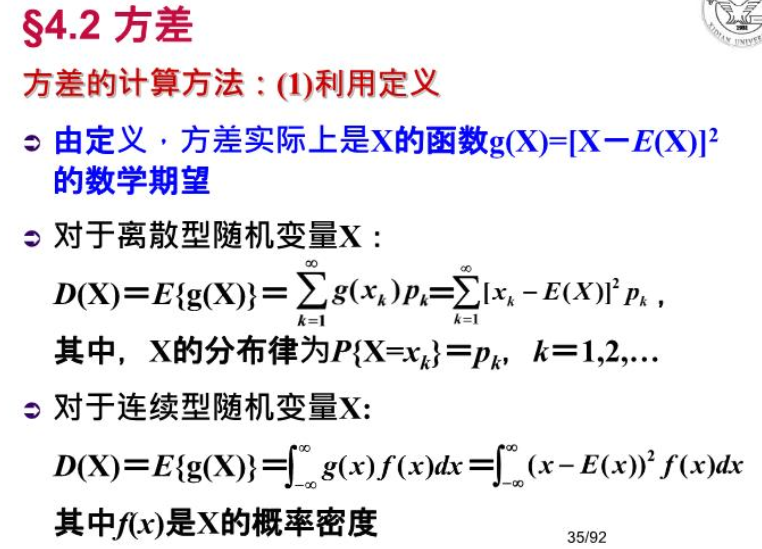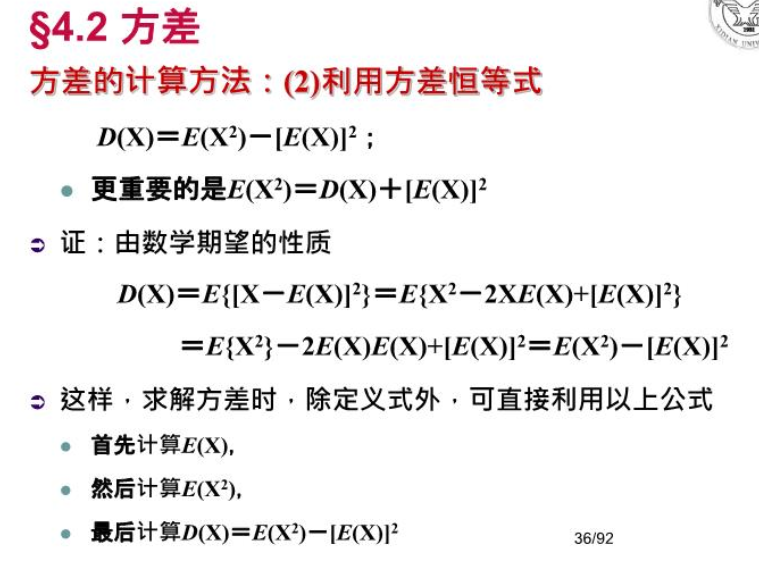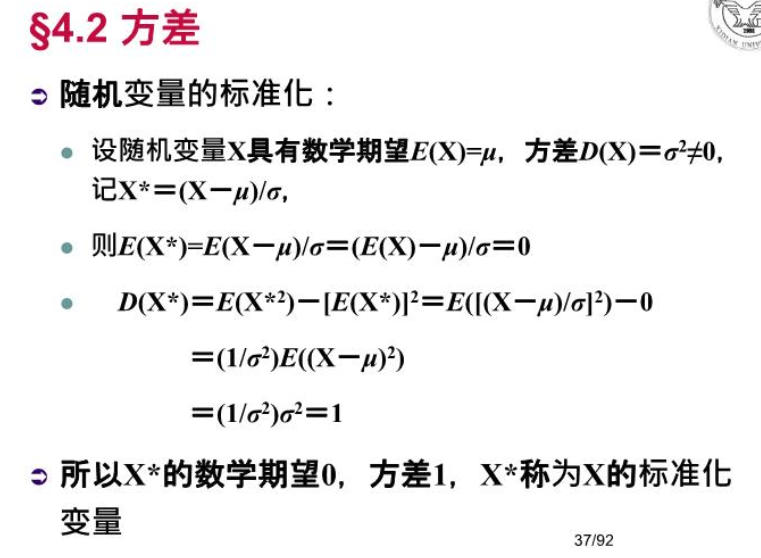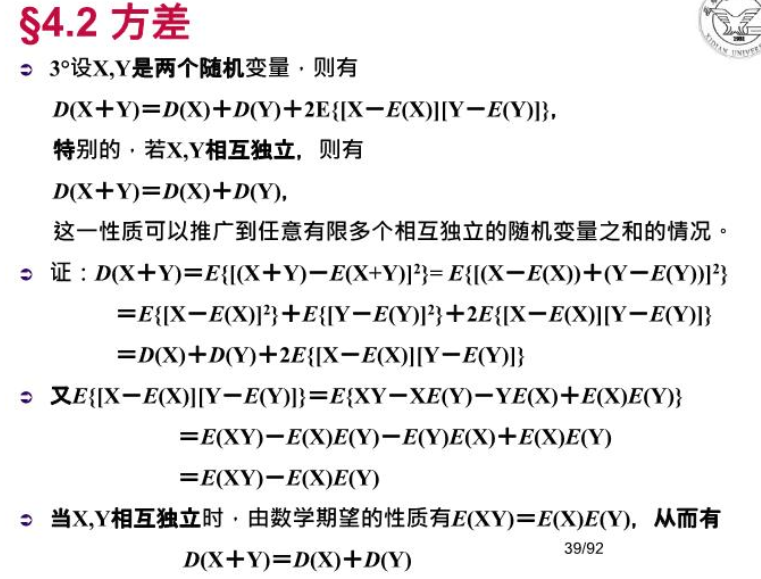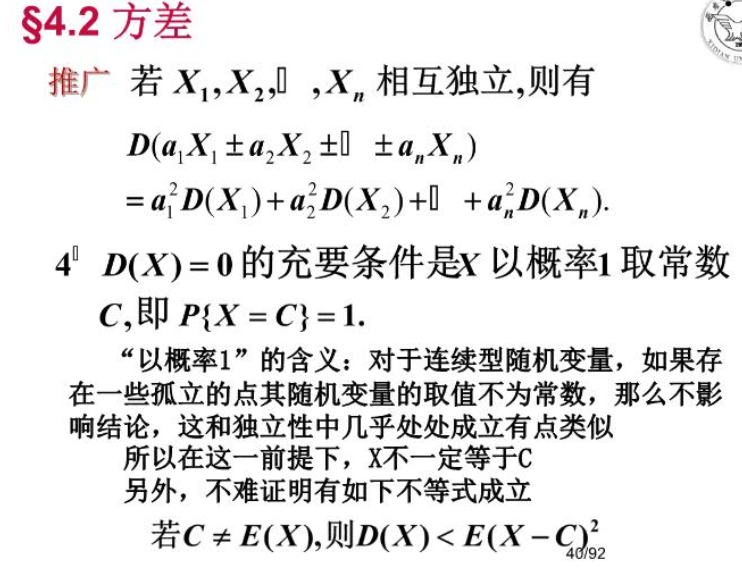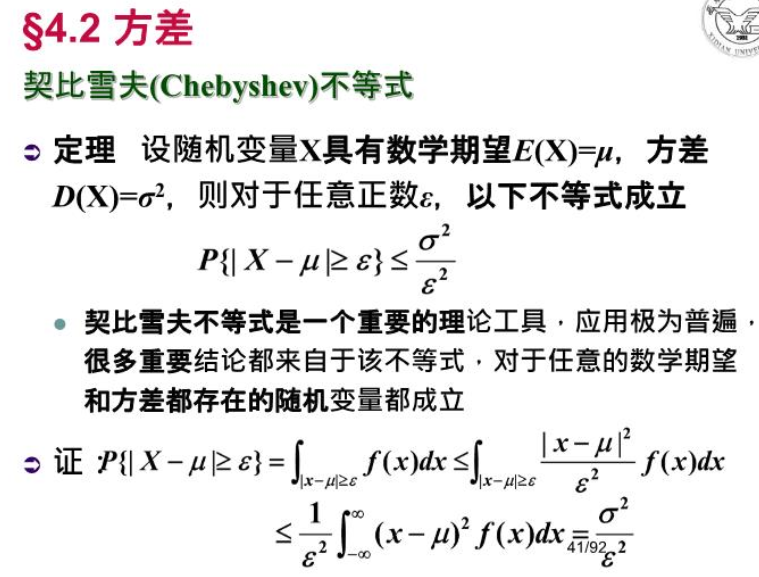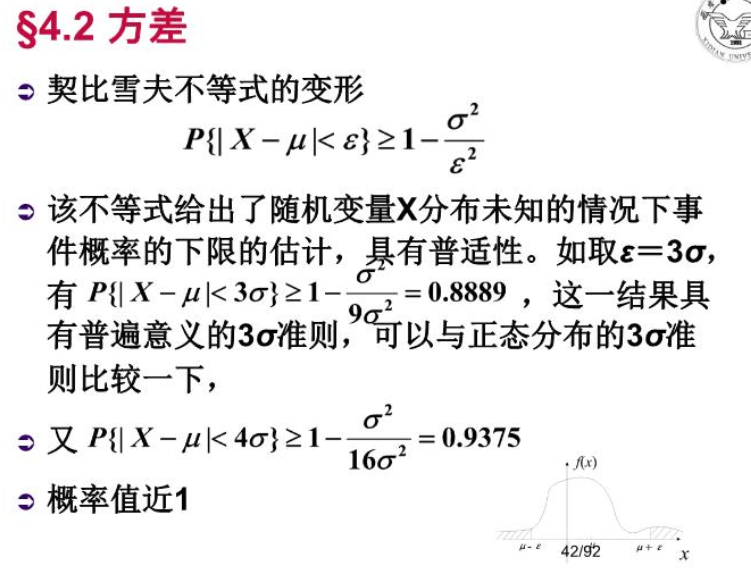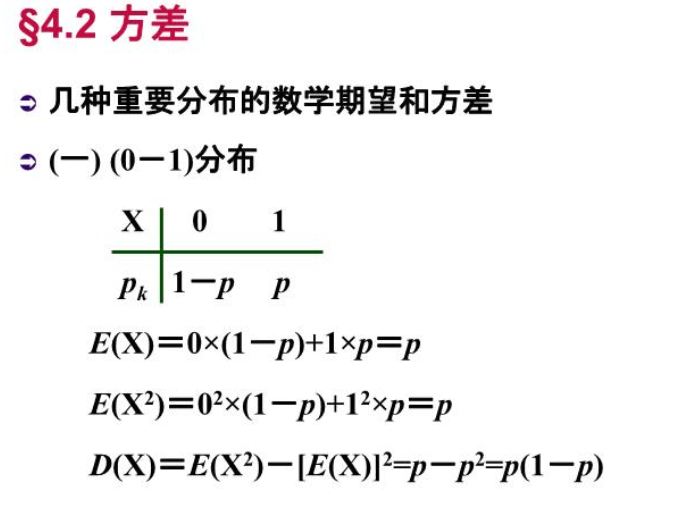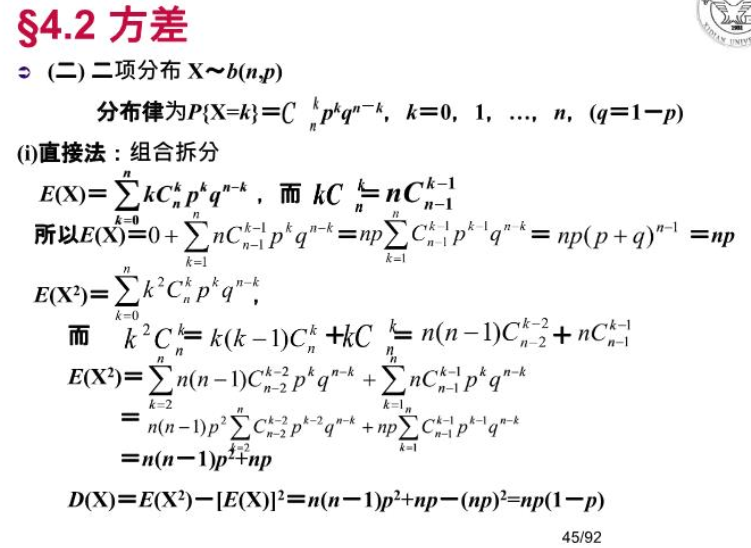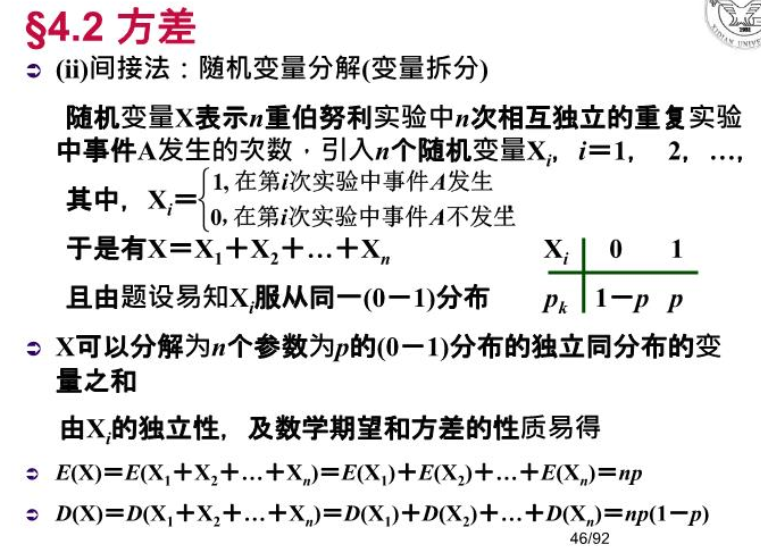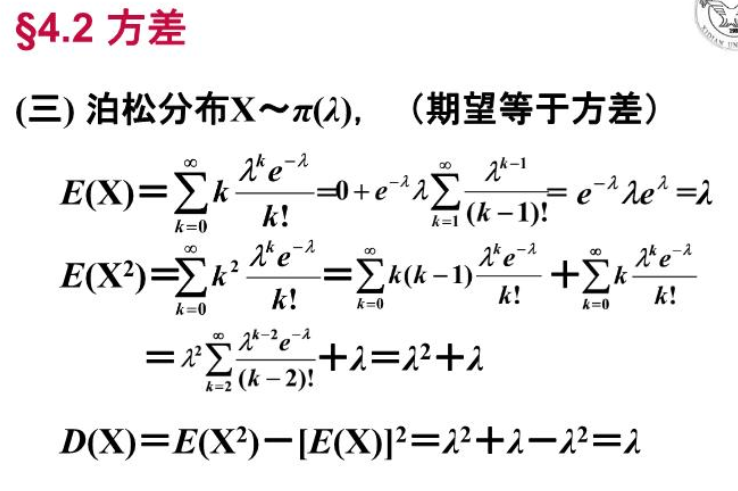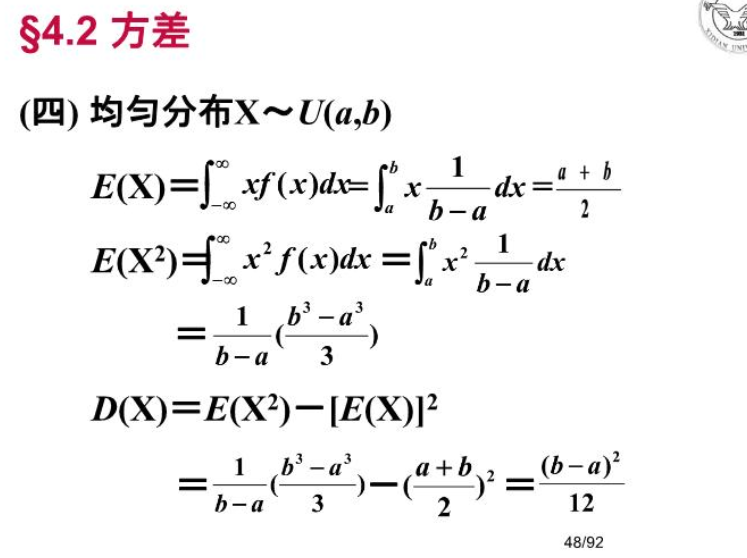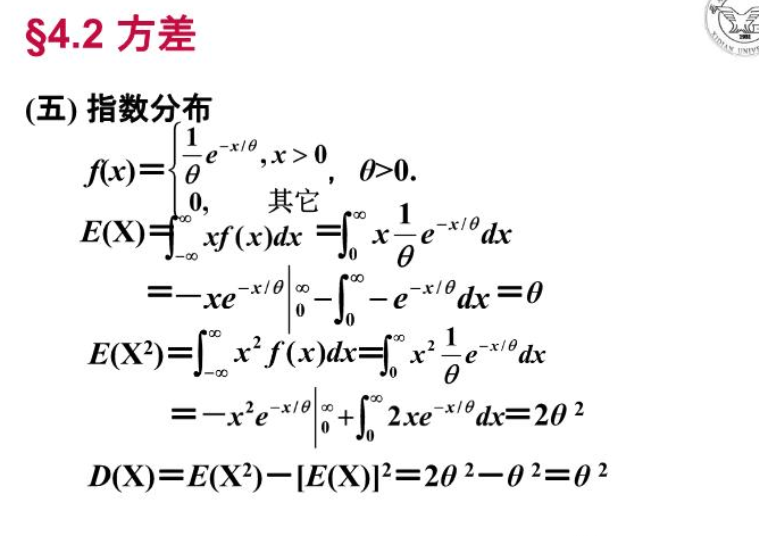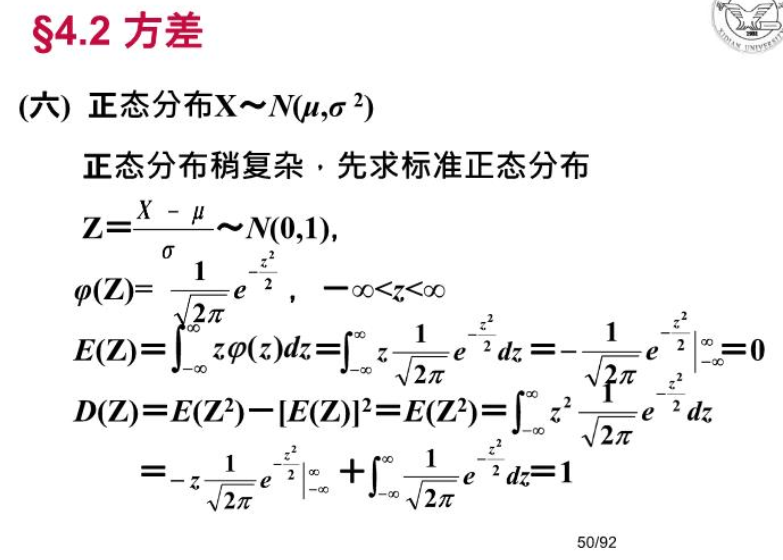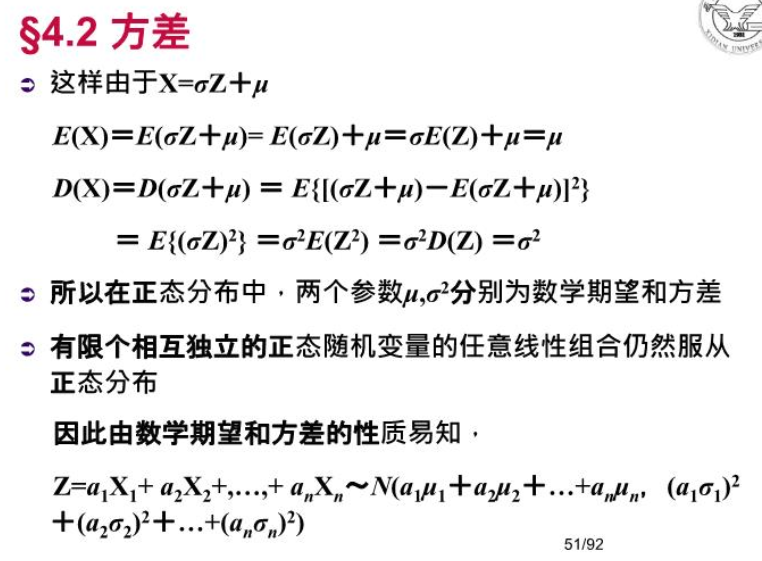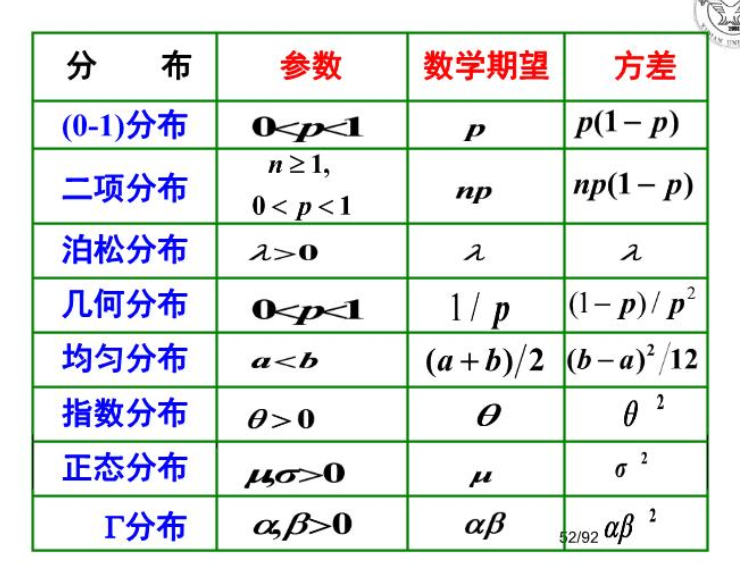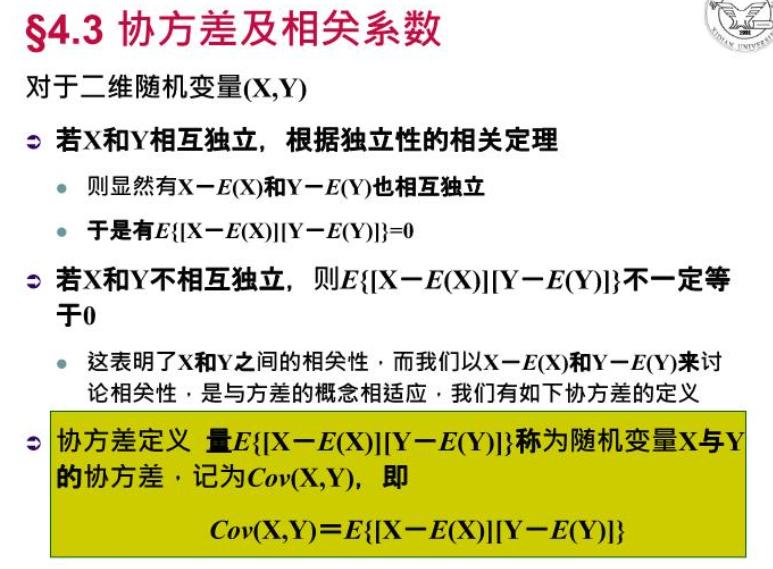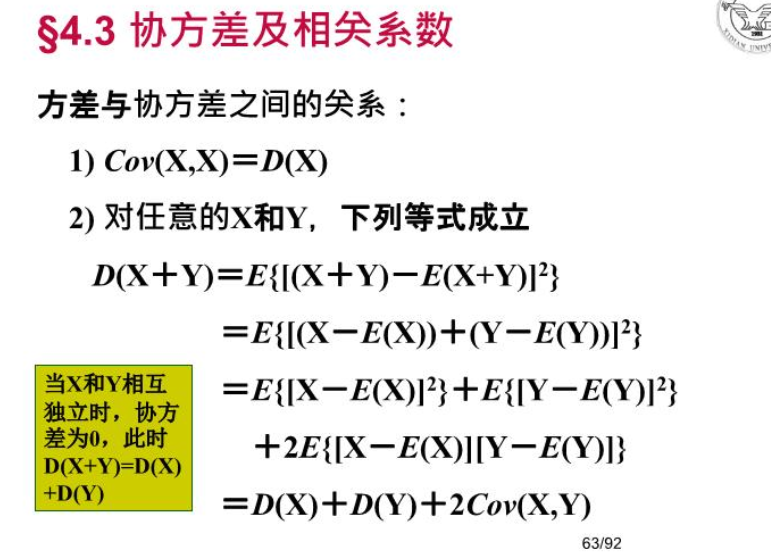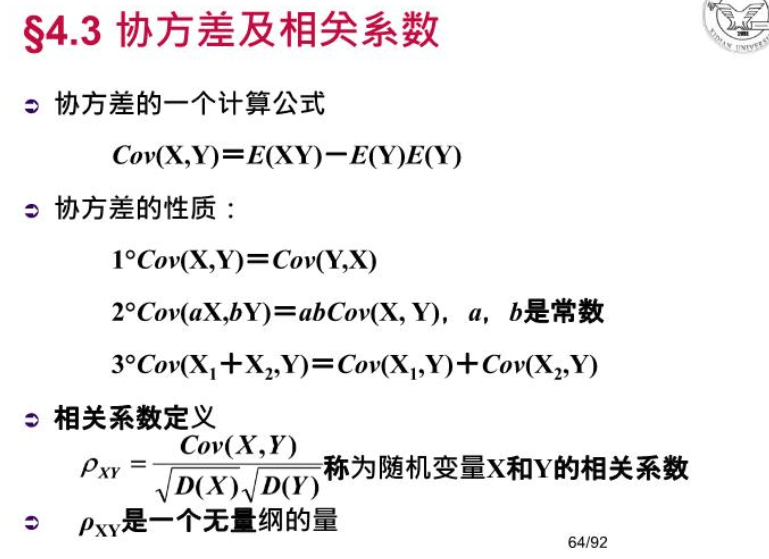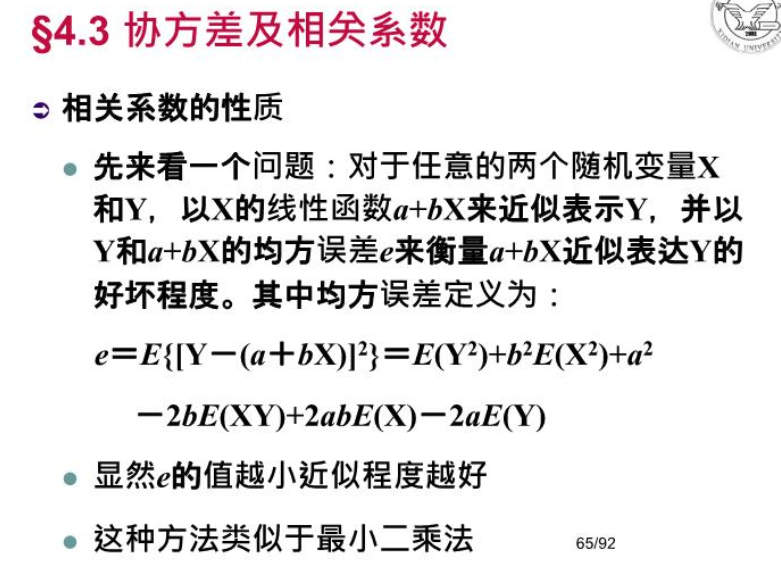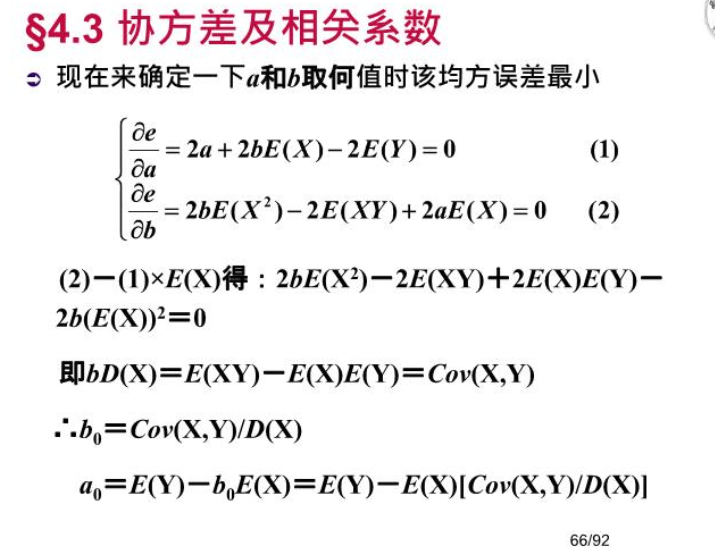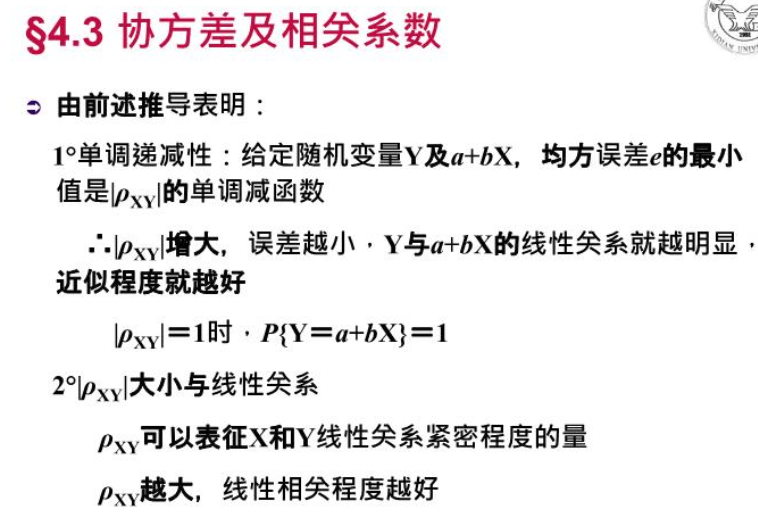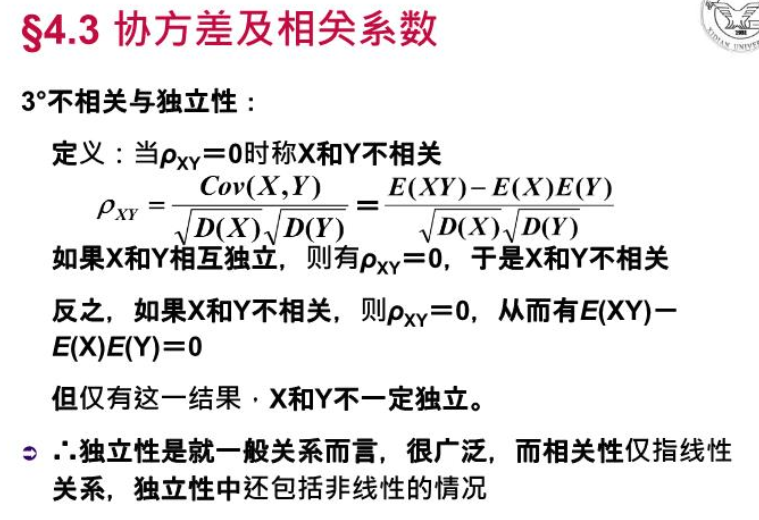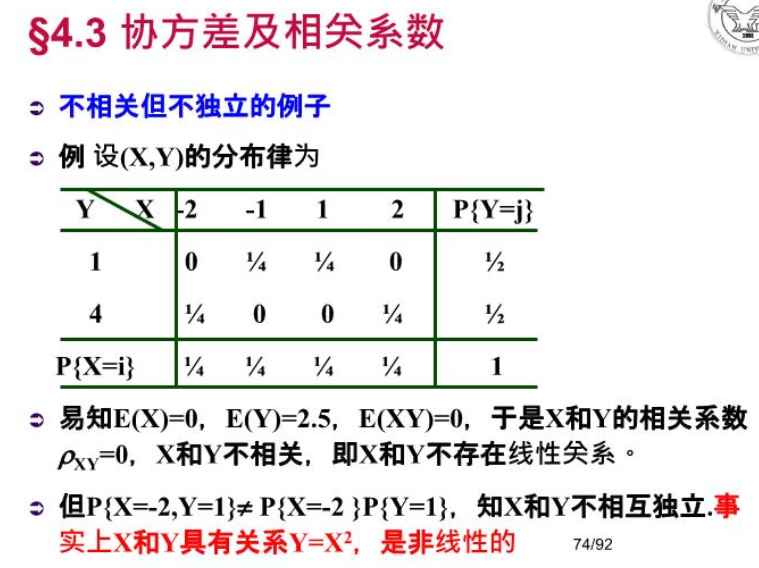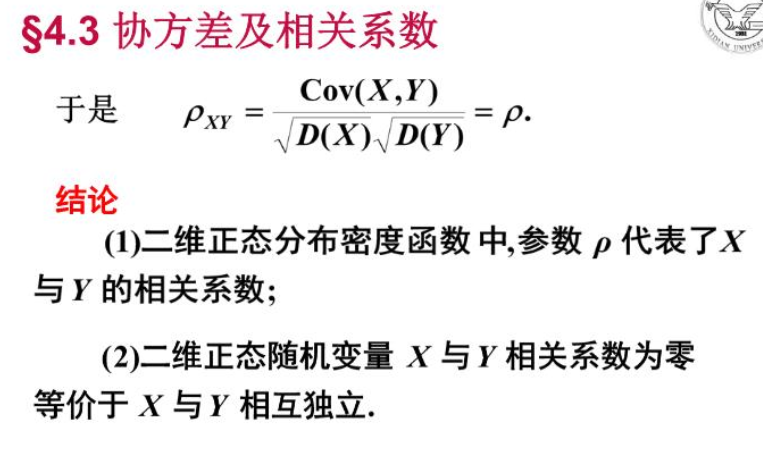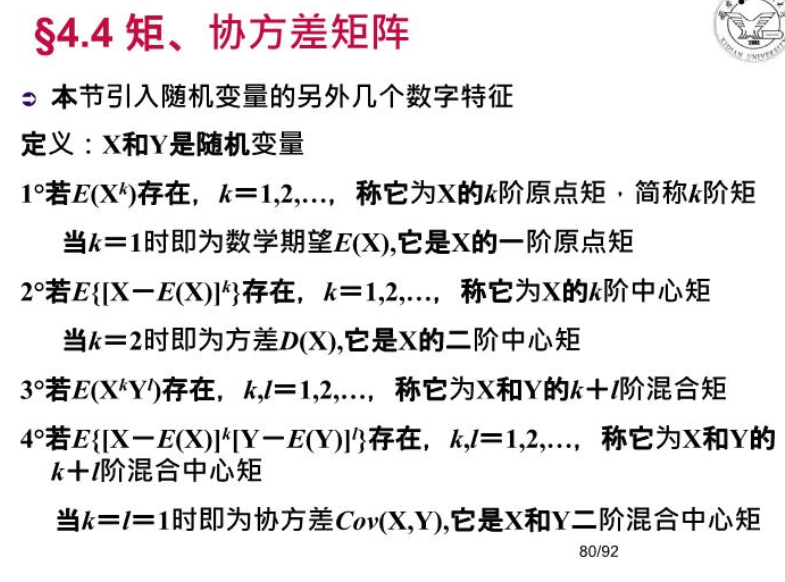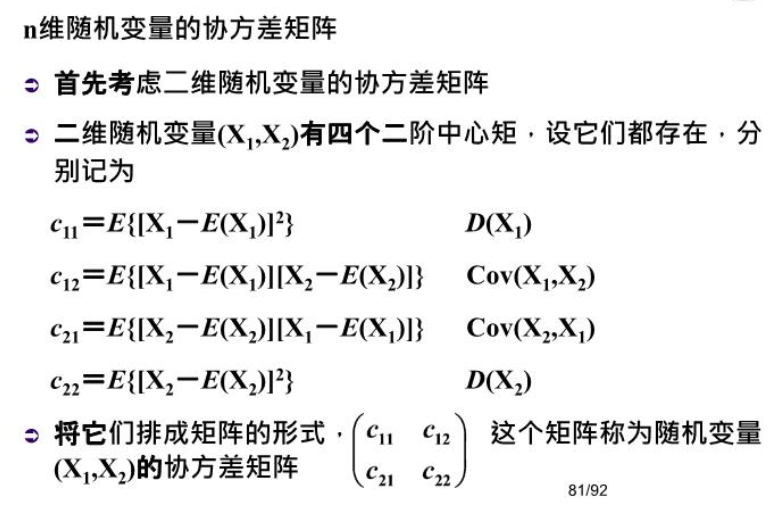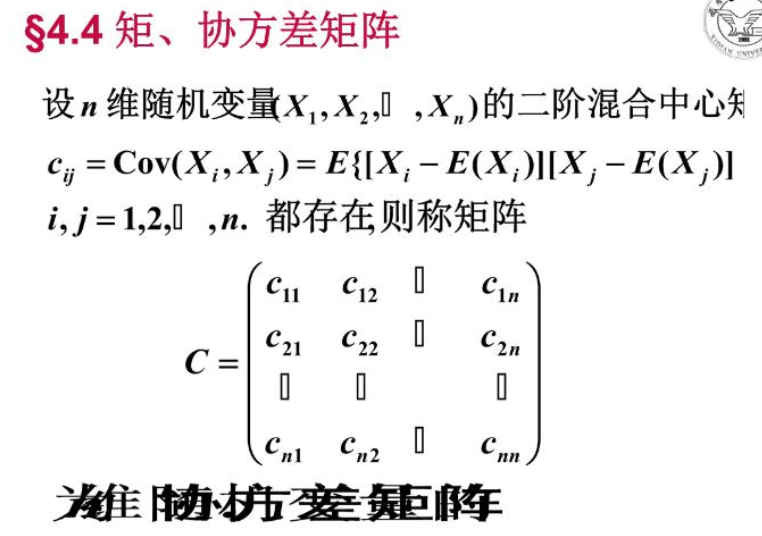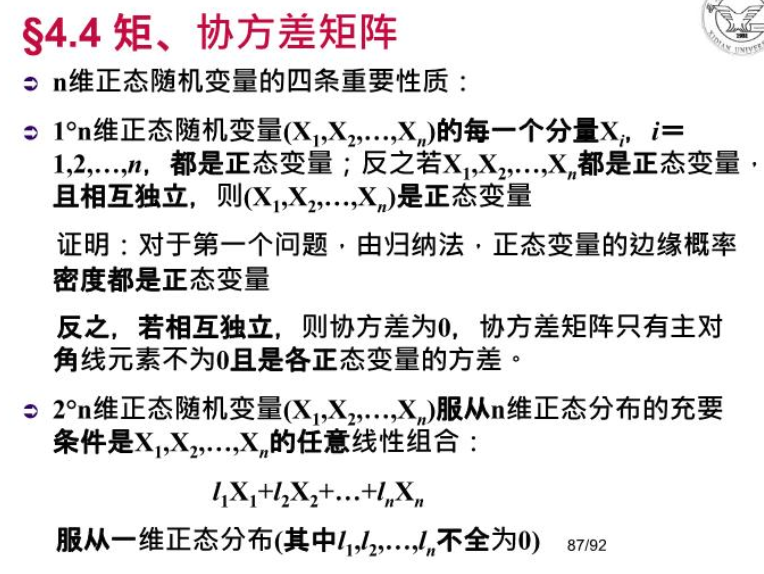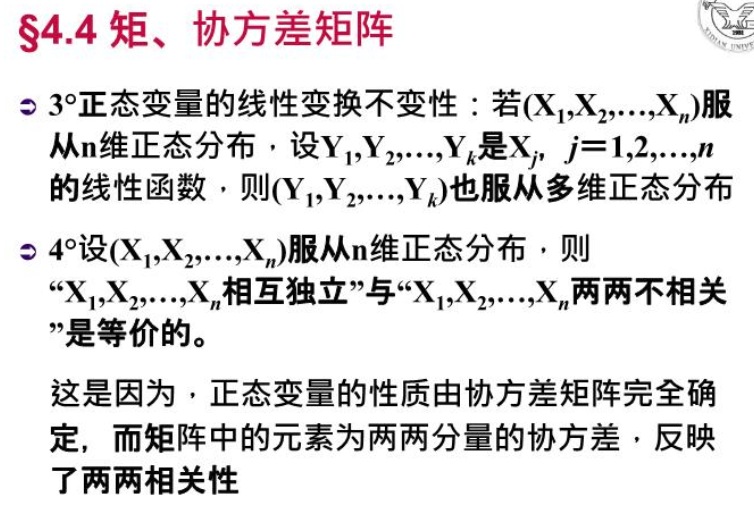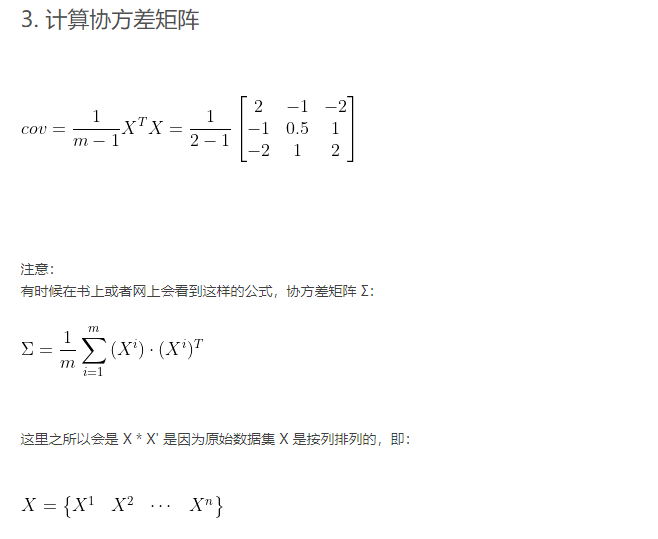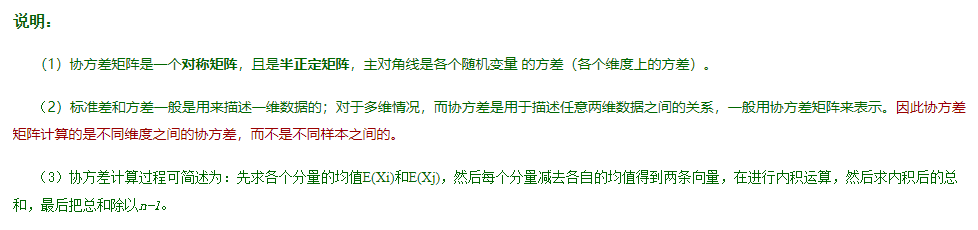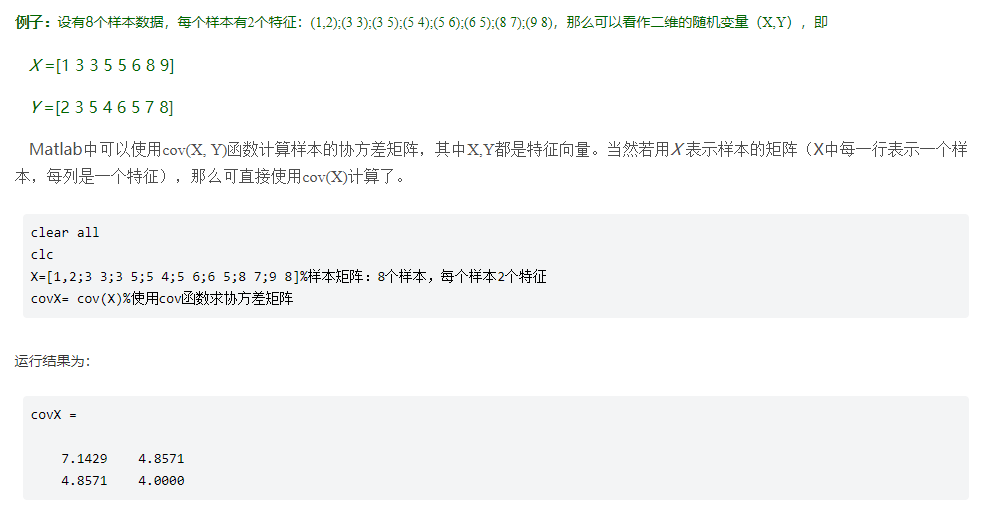散度矩阵：
散布矩阵又称散度矩阵，将协方差矩阵乘以系数（n-1）就得到了散布矩阵，所有散布矩阵与协方差矩阵矩阵的作用是一样的，理解了协方差矩阵也就理解了散布矩阵，它们只有一个系数只差而已。


展开全文• 转载出处：https://blog.csdn.net/Oxalis_Triangularis/article/details/47420521LDA中的三个散度矩阵在学习LDA（Linear Discriminate Analysis）的时候接触到了散度矩阵的概念，并且很多文章提到到混合散度矩阵等于...
•机器学习 数学
• python实现类内类间散度矩阵，并计算其商J 1 类内类间散度矩阵的数学定义 参考博客：https://blog.csdn.net/weixin_38313518/article/details/76623744 2 类内距离的理解 参考博客：...python
• def cacuSW(train_face,k):#利用总的样本矩阵计算总的类内散度矩阵 #train_face：行为样本，所有样本矩阵，每k行为一类为 m,n=train_face.shape sw=np.zeros([n,n])#大小为特征数*特征数 meanlei=np.zeros([40,n]...自然语言处理 自动驾驶 tensorflow 深度学习 神经网络
• 基于局部类内类间离散度矩阵的类别可分性判据，韩绍卿，李夕海，特征选择是模式识别领域的重要问题之一。特征选择的关键在于特征子集的评价标准。对于某些类别内呈多峰分布的特征选择问题，传统特征选择
• 这篇文章《协方差矩阵和散布矩阵（散度矩阵）的意义》讲的很透彻，分享之：http://blog.csdn.net/guyuealian/article/details/68922981 我自己补充几点自己的进一步理解： 1。通常来说特征值没有一个上限，为了...
• 转载 https://blog.csdn.net/guyuealian/article/details/68922981
• 多维随机变量的协方差矩阵，X=[X1,X2,X3,…,Xn]T，n个行向量，Xi并不取于同一样本？从n个行向量中每次取两个计算它们的协方差。 样本的协方差矩阵
• 对于二维数据集（X，Y）来说 协方差 的计算公式为：   该计算公式表明，协方差是一个数值，当X与Y如果是正相关...又叫类内散度矩阵将协方差矩阵乘以（n-1）就得到了散度矩阵散度矩阵是衡量样本分散程度的。机器学习 协方差矩阵
• Fisher线性判别中散度矩阵的表现形式可以改写,类内散度： Sw=∑i=1c∑j:yj=i(xj−μi)(xj−μi)T=12∑i,jA(w)ij(xi−xj)(xi−xj)TSw=∑i=1c∑j:yj=i(xj−μi)(xj−μi)T=12∑i,jAij(w)(xi−xj)(xi−xj)T \begin{...Fisher线性判别
• 全局散度矩阵=类内散度矩阵+类间散度矩阵 ，暂不讨论二者关系。 注意 ： 方差波动的 处理对象 只能是标量， 如果是向量数据 ，那么必须 投影成标量 然后再进行方差波动处理。 一、普通方差波动推导 SST : Sum ...LDA线性判别分析
• #train_face：行为样本，所有训练图像组成的矩阵，每个类别有k个图像为训练 sw=np.zeros([train_face.shape,train_face.shape]) meanlei=np.zeros([40,10304])#计算40个类中，每个类的平均 meanlei=[] for...机器学习 深度学习 神经网络 tensorflow python
• 【协方差】【相关系数】【协方差矩阵】【散度矩阵】均值、方差、标准差协方差为什么需要协方差协方差的通俗理解相关系数协方差矩阵概念理解Matlab协方差实战散度矩阵 均值、方差、标准差 均值： xˉ=∑i=1nxin(1) \...机器学习
• 方差 统计中的方差（样本方差）是每个样本值与全体样本值的平均数之差的平方值的平均数 概率论中方差用来度量随机变量和其数学期望（即均值）之间的偏离程度。...散度矩阵...